Next: Usage Up: Breit-Wheeler Process Previous: Breit-Wheeler Process

### Formulas

k,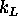,p,p'
4-momenta of the initial photon, laser photon, final electron and positron.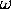,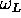,,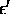Energies of the initial photon, laser photon, final electron and positron.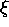Laser intensity parameter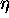Laser energy parameter: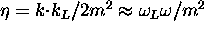.
n
Number of absorbed laser photons
x
=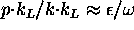. (0<x<1)
u
u=1/[4x(1-x)],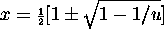, (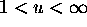).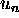Maximum u for given n: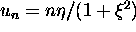.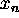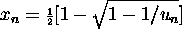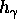Initial photon helicity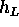Laser helicity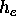Final electron helicity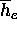`Detector' helicity of the final electron.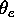Final electron angle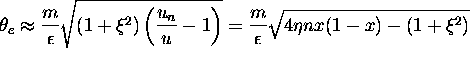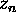The argument of the Bessel functions in the following expressions: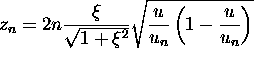Total number of pair electrons per unit time summed over the positron polarization is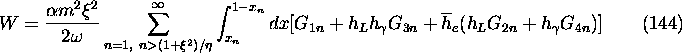Sum over final electron polarization gives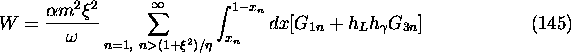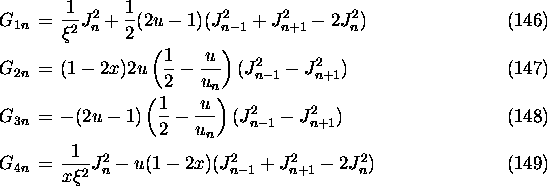These formulas can be obtained from those of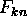by the replacement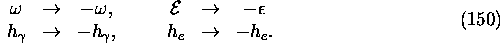This implies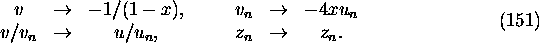For convenience, we have changed the sign as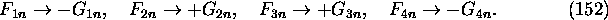For given x and n, the final momenta are given by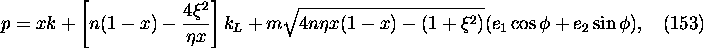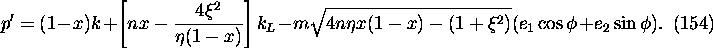Here,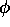is the azimuthal scattering angle in a head-on frame and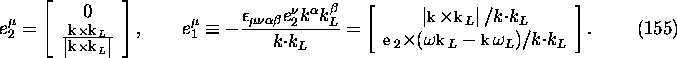These vectors satisfy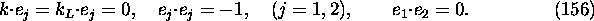Toshiaki Tauchi
Thu Dec 3 17:27:26 JST 1998